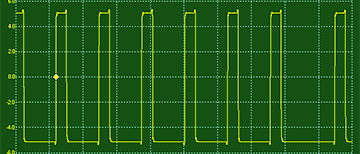### Sine-Tri Wave (variable between sine and triangle)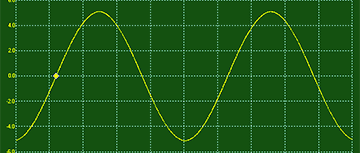### Sawtooth Wave (variable slope)### Reverse Sawtooth Wave (variable slope)### Rising Saw (variable number of teeth)### Expo (variable between expo and log curves)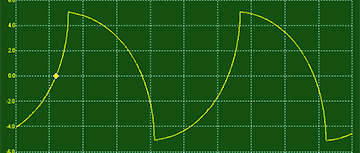### Circle Wave (variable curves)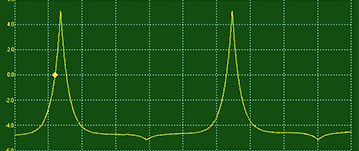### Slope Wave (variable rise and fall angle)### Pulse Wave (variable pulse width)### Stair Wave (variable step number)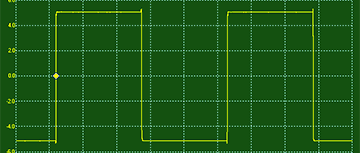### Up Down Wave (variable step number)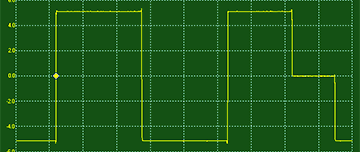### Random Wave (with variable Slew Amount)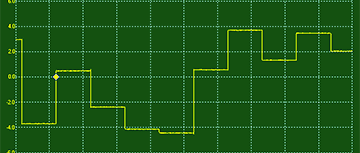### Random Cosine (variable step quantization)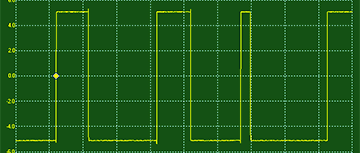### Table 1 (32 waves with smooth morphing)### Table 2 (32 waves with smooth morphing)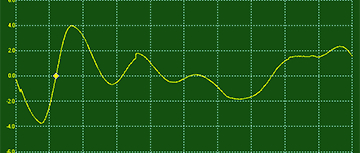### Table 3 (32 waves with smooth morphing)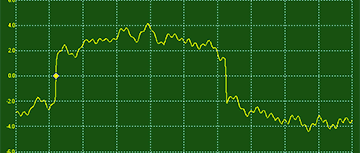### Patterns (32 rhythmic pulse patterns)